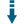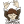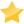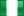Greens PHP Polygon Area: Calculate the area and perimeter of a polygonDownload .zip
 InfoExampleView files (6)Download .zip Reputation Support forum Blog Links
 Ratings Unique User Downloads Download Rankings47% Total: 270 All time: 7,640 This week: 175Version License PHP version Categories greens-polygon 1.0.0 GNU General Publi... 5 Algorithms, PHP 5, Geography, Math
Description Author

This class can calculate the area and perimeter of a polygon.

It implements the Green's theorem to calculate the area of an irregular polygonal shape given the coordinates of the vertices.

The class can also calculate the perimeter of the polygon.

Recommendations

Area of polygon in Google Maps
Calculate the area of polygon drawn in a map

Area of a polygon
How to calculate the area of a polygon?

Innovation AwardSeptember 2015 Number 6Prize: One year server license IP to country, region, city, latitude, longitude, ZIP code, time zone, area code database Calculating the area of regions on the map is important for instance to determine the value of a land property. However, when the land shape is not rectangular, it is not trivial to compute the property area. This class can compute the area of irregular polygons that form regions on the map using the Greens theorem. Manuel LemosPerformance Level
Name: Classes: Samuel Adeshina is available for providing paid consulting. Contact Samuel Adeshina . 19 packages by Samuel AdeshinaNigeria 23 540 2 in Nigeria1065 in NigeriaInnovation awardNominee: 7x

Winner: 1x

Details

How To Instantiate This Class

It determines the area and perimeter of a polygon by accepting the vertices (_coordinates_) of the polygon. _These parameters can be provided in three different ways_: 1. Supplying them in the constructor definition You can do this by adding the coordinates as a Point object as parameter.

A Point Object is a new instance of a point class that's already been included in the polygon.class.php class file. You can find the source code of this class in the point.class.php class file To provide the vertices of the polygon as parameters of the constructor, _you may instantiate it this way_:

`\$polygonObjectInstance = new Polygon(new Point(0, 0), new Point(2), ...);`

The constructor accepts as many parameters as is possible, _although, they're all optional_ 2. Providing them as parameters to the setVertice() method The setVertice method accepts a single parameter which must be an instance of the Point object. This parameter acts as the coordinate/vertex of a polygon

`....`
`\$pointObjectInstance->setVertice(new Point());`
`\$pointObjectInstance->setVertice(new Point(2, 3));`
`//This option provides a coordinate with the x and y axis at the (0, 0) and (2, 3) positions on a plane`
1. Providing them as parameters to the setVertices() method Unlike the setVertice method, this method accepts more than one parameter just like the constructor but they all do the same thing, to populate an array with a set of coordinates.

You can get both the area and parameter by calling either of the area() or perimeter() methods respectivelyFiles
File Role DescriptionHow-To.md Doc. A quick tutorialLICENSE Data License Filepoint.class.php Class Point Classpolygon.class.php Class Polygon ClassREADME.md Data Read Metest.php Example Examples And How To

 All time: 7,640 This week: 175All time Utility: 58%58%66%58%- - 47%2583Advertise on this siteSite mapNewsletterStatisticsSite tipsPrivacy policyContact